CTET Quants Questions

## CTET Quants QuestionsJobs By Batch
Jobs By Location
Jobs By Degree
Jobs By Branch
IT Jobs
Internships
Govt. Jobs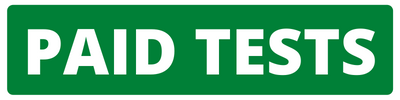• ##### Original Questions of IT Companies| All Topics### Quiz Begins Here

Q #1
:

#### Quants Question

Directions: These questions are based on the bar graph, which gives the production and sales of trinkets in India, for the period 1991 to 1994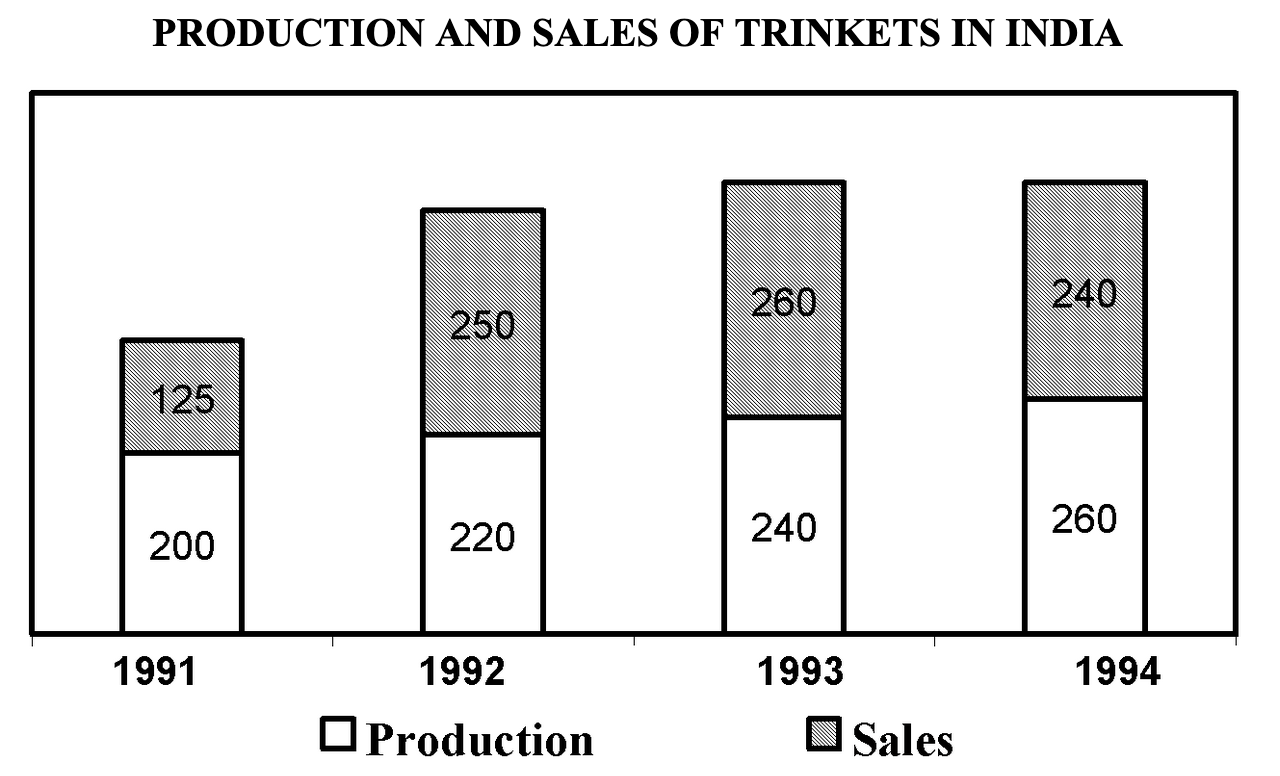Assuming there was no stock of trinkets in India at the beginning of 1991, which of the following statements is true for 1994?
+

Explanation: Since we do not know whether the excesses each year on exported or not, we cannot comment regarding 1994.

Q #2
:

#### Quants Question

Directions: These questions are based on the following table, which gives the fatality and accident rates in coal mines in India for the period 1973 to 1989.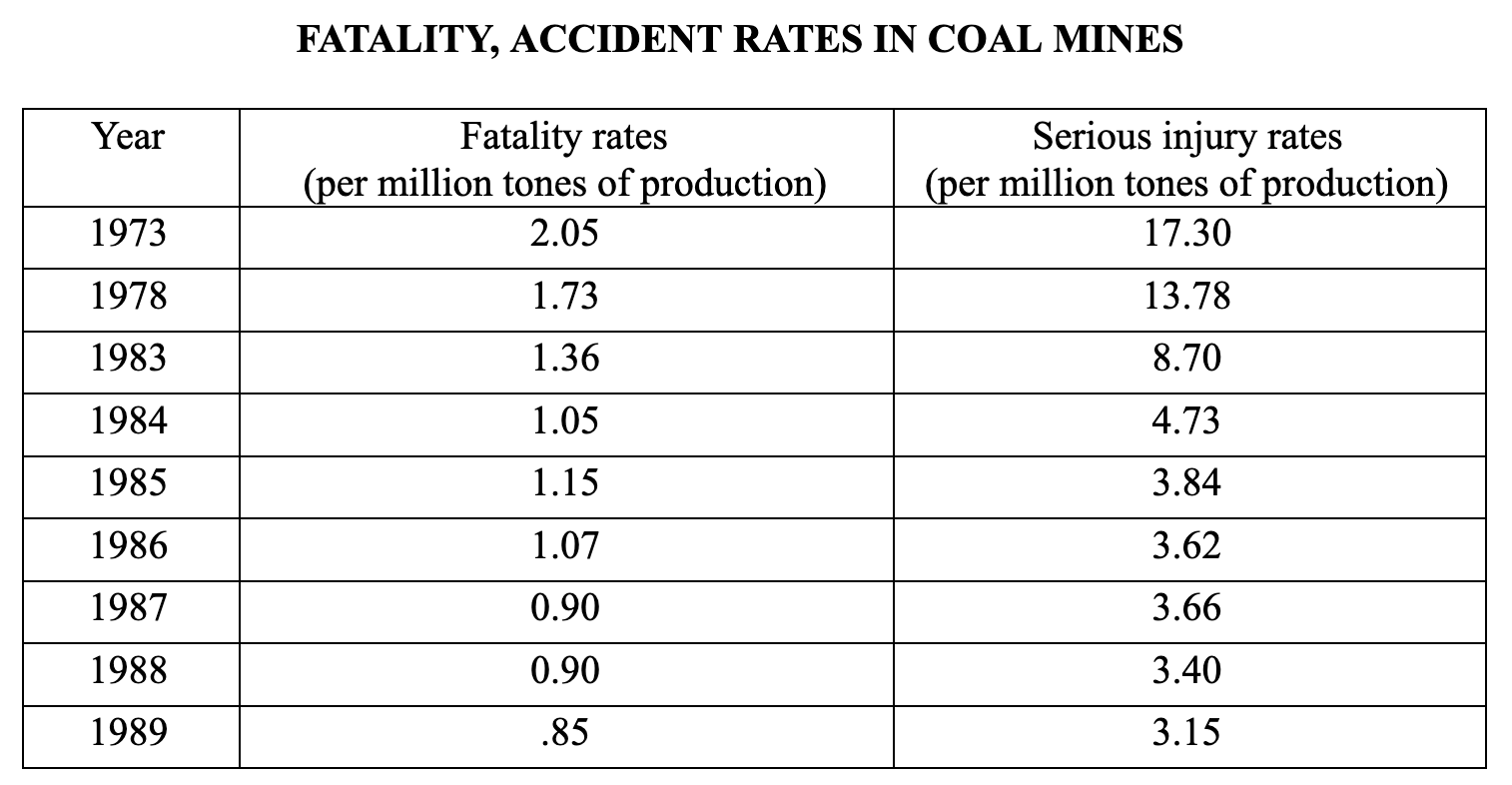The figures given above were released on December 1, 1989. After the figures were released, 5% of the seriously injured workers died. What is the resultant percentage increase that the fatalities figure for that year should undergo, assuming there were no fresh accidents in December 1989?
+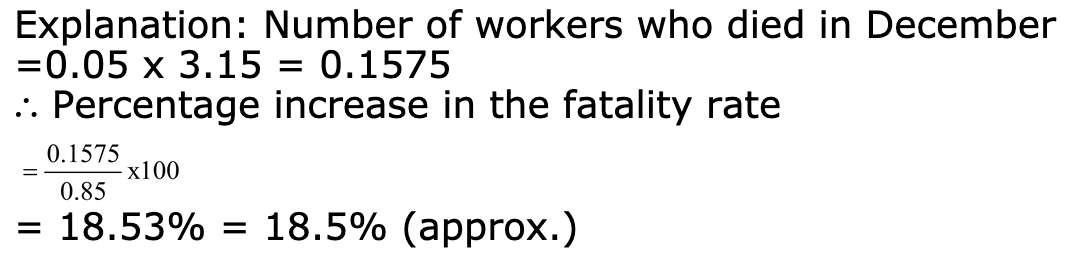Q #3
:

#### Quants Question

Directions: These questions are based on the line graph given below which gives the number of graduates passing out of Indian Universities (x), the number of graduates getting U.S. visa (Y) and the number of students applying for Indian Management Institutes (Z).The Value of Z in 1990 is 35000.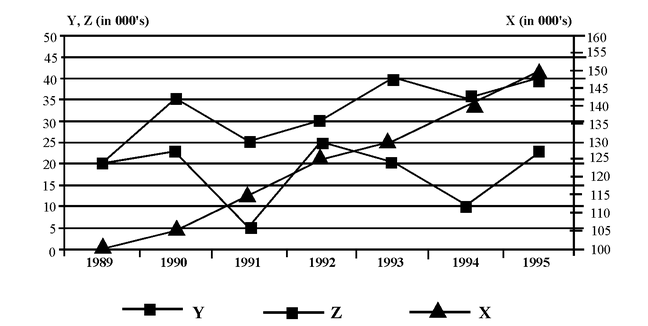If it is believed that the relationship between X, Y and Z is best expressed by Z = X - 4Y for any year, the data for which of the following years best satisfies this relationship?
+

Explanation: In 1989, Z = 20, Y = 20 X = 100 Z = X – 4Y.

Q #4
:

#### Quants Question

Directions: These questions are based on the information and table given below.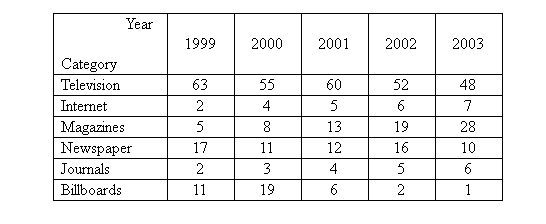India has witnessed a tremendous growth in terms of the media industry. This growth has also resulted in a huge increase in the advertising revenue of various categories of media. The following table shows the percentage share of total revenue earned by each media of the total advertising revenue of the industry from the years 1999 to 2003. The total revenue of the industry in 2000 was lower than that in 2002 while the revenue in 2001 was higher than that in 2003.

The percentage share of revenue of which of the following categories was increasing at an increasing rate?
+

Explanation: By observation we can see that only Internet, Magazines and Journals have been increasing but of these internet and journals are increasing at a steady rate whereas Magazines is increasing at an increasing rate.

Q #5
:

#### Quants Question

DIRECTIONS: are based on the following graph: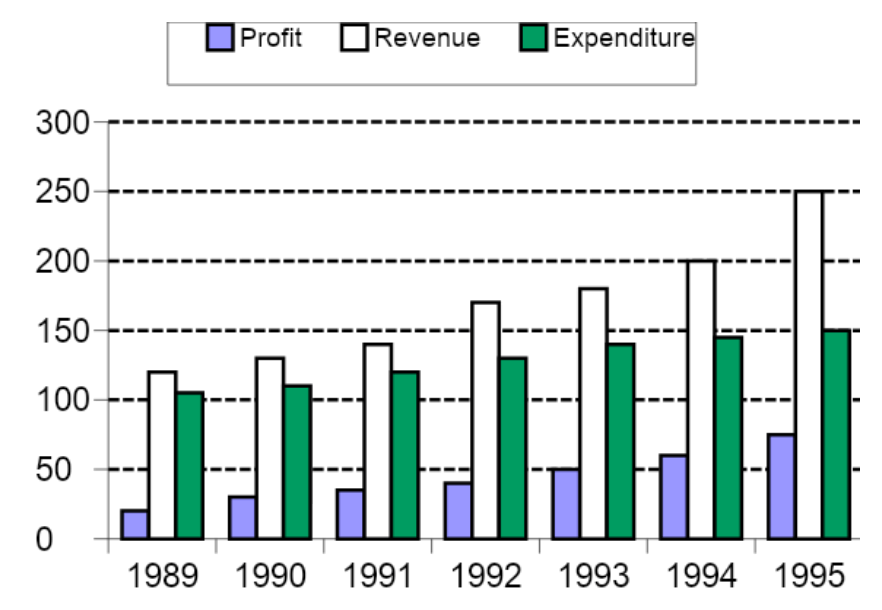The expenditure for the seven years together form what percent of the revenues during the same period ?
+

Explanation: Total Revenue = 1174 Total Expenditure = 700 + (2 + 5 + 15 + 30 + 35 + 40 + 50) = 887 %formed by the revenue = 887/1174 ~ 900/1200 = ¾ = 75% [Actual values will give 75.55% & again (1) will be the correct answer but you can save time using approximations]